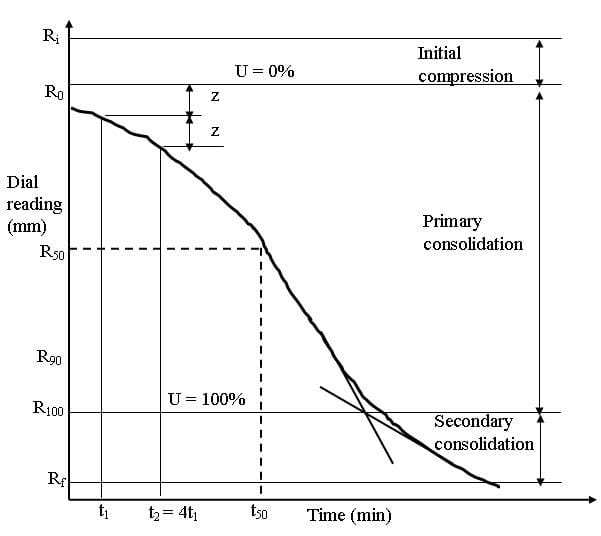Casagrande’s Logarithm of Time Fitting Method- Determination of Coefficient of Consolidation of Soil

# Casagrande’s Logarithm of Time Fitting Method- Determination of Coefficient of Consolidation of Soil - Notes | Study Soil Mechanics Notes- Agricultural Engineering - Agricultural Engineering

 1 Crore+ students have signed up on EduRev. Have you?

Casagrande’s Logarithm of Time Fitting Method

From the oedometer test (explained in Lesson 23) the dial reading (settlement) corresponding to a particular time is measured.  From the measured data, dial reading vs time graph can be drawn (as shown in Figure 24.2). Select two points t1 and t2 in initial part of the curve such that t= 4 t1. The points corresponding to the chosen times are marked on the curve. The vertical distance (z) between the two points on the curve is measured. Select another point R0 such that the vertical distance between that point and point on the curve corresponding to the ttime is also z. R is corrected zero reading i.e U = 0%. Determine the U=100% line by drawing two tangents form the straight portion of the curve as shown in Figure 24.2. Once U=0% and 100% lines are identified, U= 50% line is also determined by choosing the middle point between the U=0% and 100% lines. Time (t50) corresponding to the 50% degree of consolidation is determined from the curve. The Coefficient of consolidation (cv) is determined from Eq.(24.1).Fig. 24.2. Casagrande’s Logarithm of Time Fitting Method.

The document Casagrande’s Logarithm of Time Fitting Method- Determination of Coefficient of Consolidation of Soil - Notes | Study Soil Mechanics Notes- Agricultural Engineering - Agricultural Engineering is a part of the Agricultural Engineering Course Soil Mechanics Notes- Agricultural Engineering.
All you need of Agricultural Engineering at this link: Agricultural Engineering

## Soil Mechanics Notes- Agricultural Engineering

64 docs
 Use Code STAYHOME200 and get INR 200 additional OFF

## Soil Mechanics Notes- Agricultural Engineering

64 docs

Track your progress, build streaks, highlight & save important lessons and more!

,

,

,

,

,

,

,

,

,

,

,

,

,

,

,

,

,

,

,

,

,

;